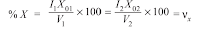WAZIPOINT Engineering Science & Technology: Transformer: Percentage Resistance, Reactance, and Impedance

## Thursday, February 2, 2023

### Transformer: Percentage Resistance, Reactance, and Impedance

The quantities Percentage Resistance, Reactance, and Impedance of a transformer are usually measured by the voltage drop at full-load current expressed as a percentage of the normal voltage of the winding on which calculations are made.

### (i) Percentage resistance at full-load

The percentage resistance and reactance of a transformer are 2% and 4% respectively. The approximate regulation on full load at 0.8 pf lag

### (ii) Percentage reactance at full-loadThe reactance of the transformer is nothing but leakage reactance of the transformer. This phenomenon in transformers is known as Magnetic leakage. Voltage drops in the windings occur the due to impedance of the transformer. Impedance is the combination of resistance and leakage reactance of the transformer.

### (iii) Percentage impedance at full-load

The percentage impedance of a transformer is the volt drop on full load due to the winding resistance and leakage reactance expressed as a percentage of the rated voltage. It is also the percentage of the normal terminal voltage required to circulate full-load current under short circuit conditions.

It should be noted from above that the reactance and resistances in ohm can be obtained thus :

It may be noted that percentage resistance, reactance, and impedance have the same value whether referred to as primary or secondary.

You may know the details about the electrical transformer from the following articles: